[Next] [Up] [Previous]

# Heptagonal Tilings

Tilings with heptagonal symmetry require a fractal acceptance domain if produced by the projection method, but they can be produced by dualization as easily as tilings with other symmetries. However, if we start simply, avoiding advanced mathematics, and just use heptagons in an attempt to obtain a Keplerian tiling, we may start with something like this: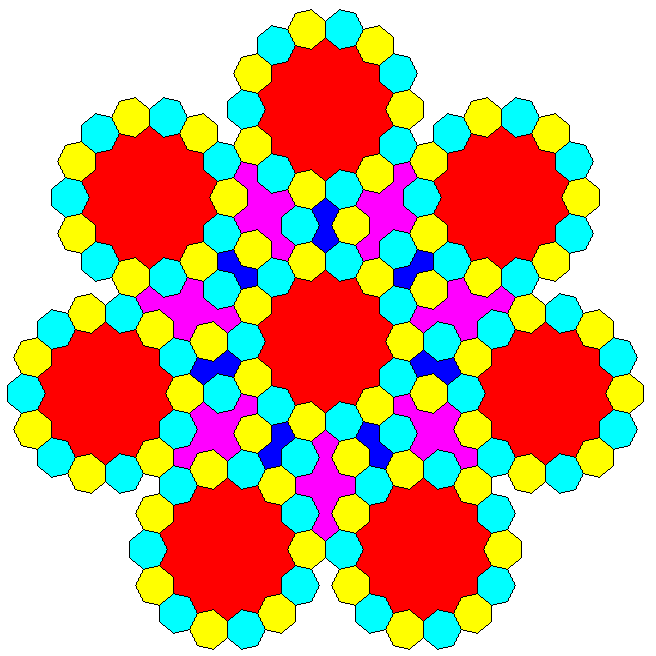This can be extended easily enough to produce a tiling of the Dürer type, where seven flattened hexagonal tilings built from heptagonal shapes meet at a sevenfold symmetric centre: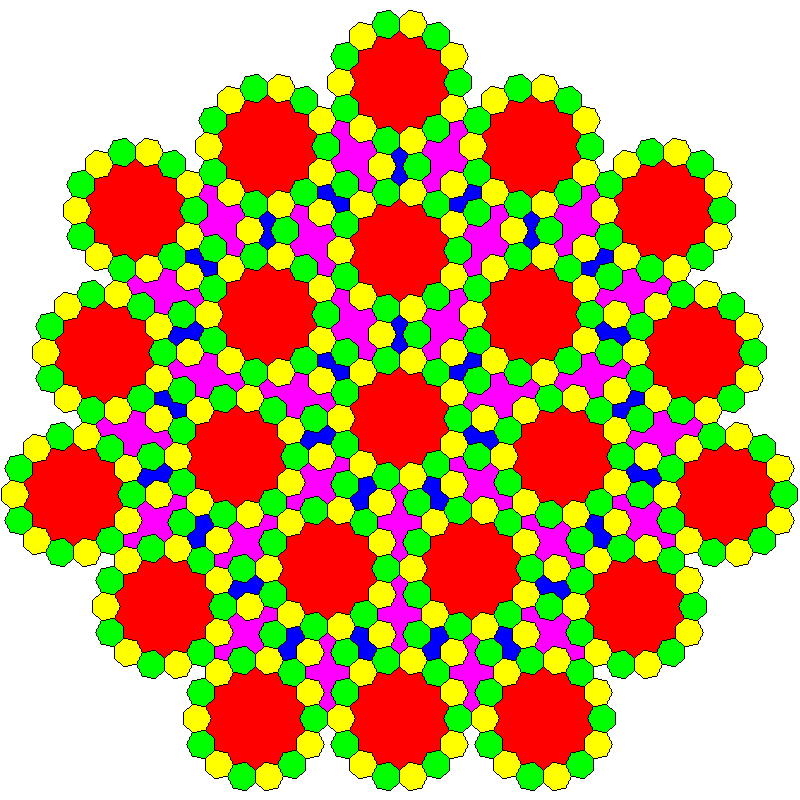but achieving a recurrence relation, so that one doesn't simply have a conventional symmetry twinned, which is fundamentally trivial, but instead an intrinsic global sevenfold symmetry (of the limited-range sort that is the only one possible) is more difficult.

The existing heptagonal tilings are, instead, based on rhombs. Of the two shapes shown below, the one on the right, most often without its outer shell of thin rhombs, is the one that is found in the heptagonal tilings generated by the standard mathematical method of dualization, also called the grid method.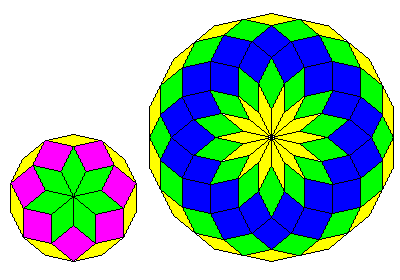But these tilings do also have spaces suitable for placing the symmetric shape on the left as well. One might begin to create a recurrence relation inspired by these two shapes, following the principle of a binary tiling, as follows: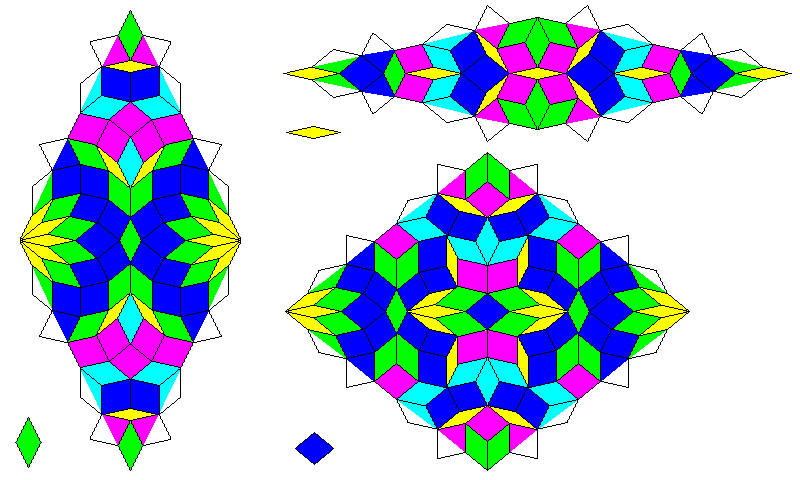but continuing to create a complete recurrence, creating the recurrences for tiles whose borders run in the other direction, indicated by different colors, purple instead of blue and blue-green instead of green runs into problems.

But it is still possible to create a tiling with heptagonal symmetry from this incomplete recurrence. If one is prepared to chop the rhombs in half, to create triangles, then the direction in which the borders run is no longer a concern for the recurrence relation. Tilings with heptagonal symmetry which use only the rhomb shapes, and no triangles, definitely do exist. For example, a paper by E. O. Harriss gives this recurrence rule for one: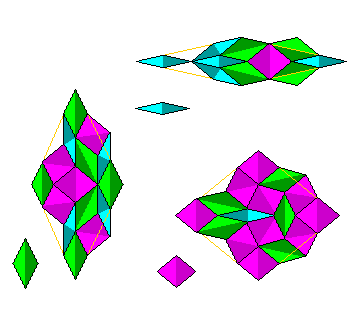but the resulting tiling does not give heptagonal symmetry in as unmistakably obvious a form as one might like.

The first substitution tiling with sevenfold symmetry was based on triangles, instead of rhombs; it was by L. Danzer, but his best known tiling is a three-dimensional one with icosahedral symmetry.

[Next] [Up] [Previous]